## Transmission Line Formulas

 Concentric Transmission Lines Characteristic impedance in ohms is given by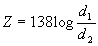R-f resistance in ohms per foot of copper line, is given by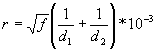Attenuation in decibels per foot of line, is given by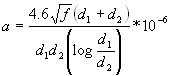Where: Z r   a d1   d2   f = =   = =   =   = characteristic impedance in ohms, radio frequency resistance in ohms per foot of copper line, attenuation in decibels per foot of line, the inside diameter of the outer conductor, expressed in inches, the outside diameter of the inner conductor, expressed in inches, frequency in megacycles. Two-Wire Open Air Transmission Lines Characteristic impedance in ohms is given by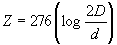Inductance in micro henrys per foot of line is given by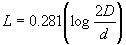Capacitance in micromicrofarads per foot of line is given byAttenuation in decibels per foot of wire is given byR-f resistance in Ohms per loop-foot of wire, is given by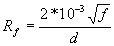Where: Z D d L C db Rf f = = = = = = = = characteristic impedance in ohms, spacing between wire centers in inches, the diameter of the conductors in inches, inductance in microhenrys per foot of line,capacitance in micromicrofarads per foot of line, attenuation in decibels per foot of wire, r-f resistance in ohms per loop-foot of wire,frequency in megacycles. Vertical Antenna The capacitance of a vertical antenna, shorter than one-quarter wave length at its operating frequency, is given by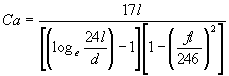Where: Ca l d f e = = = = = capacitance og the antenna in micromicrofarads, height of antenna in feet, diameter of antenna conductor in inches, frequency in megacycles. 2.718 (the base of the natural system of logarithms).